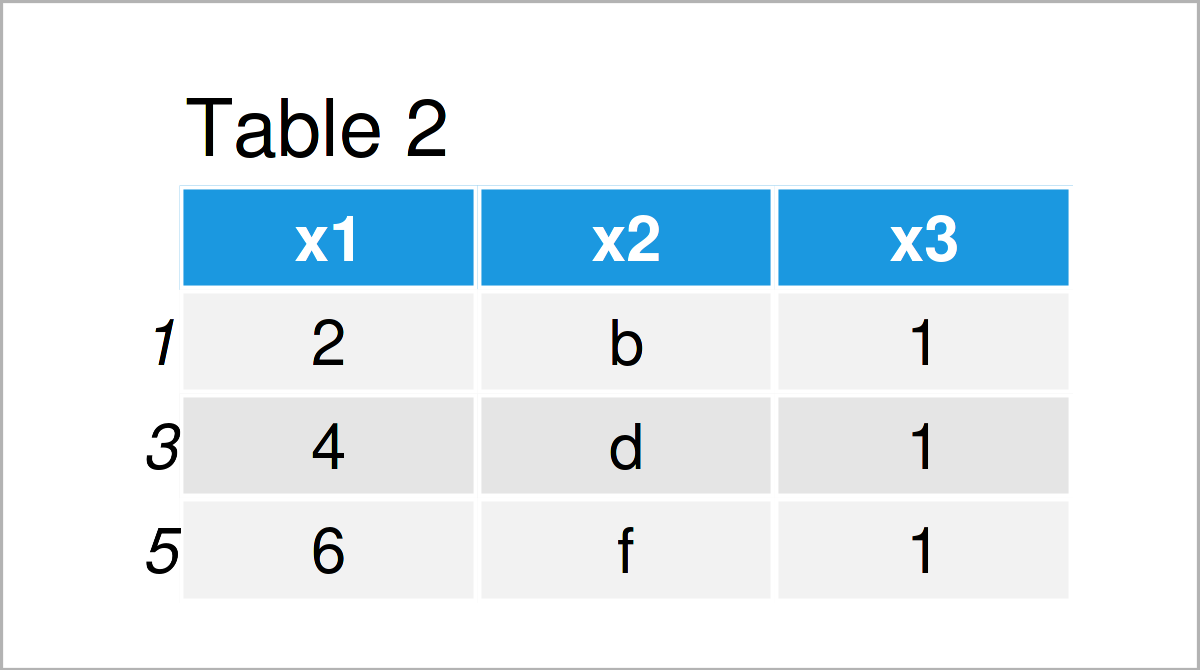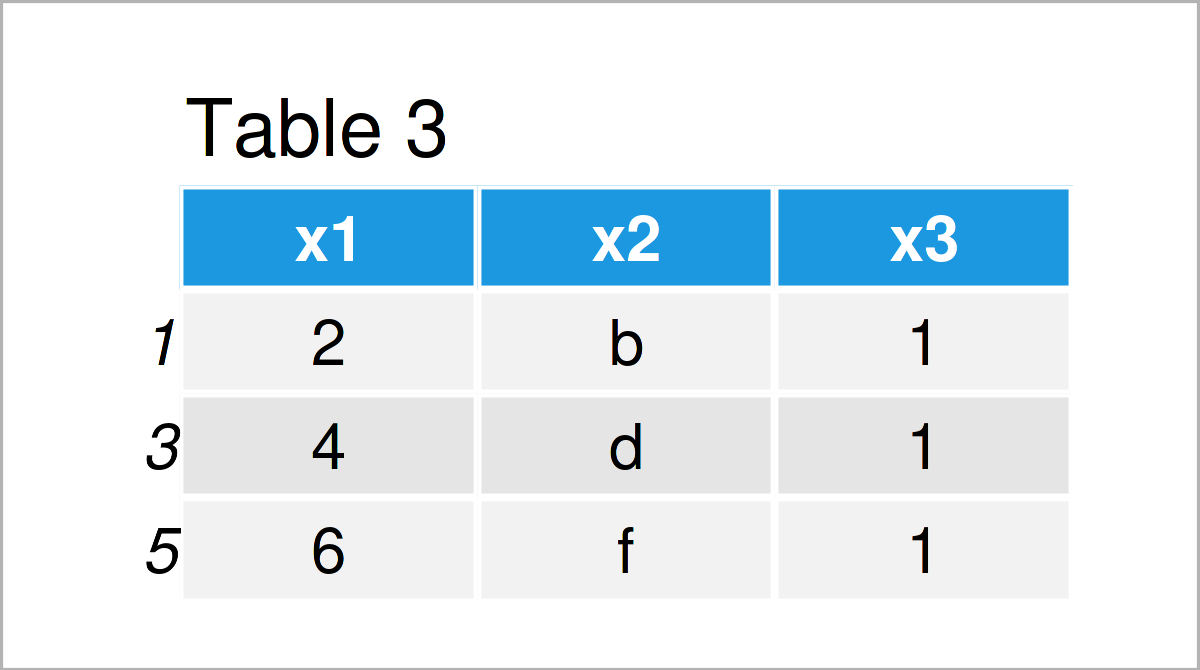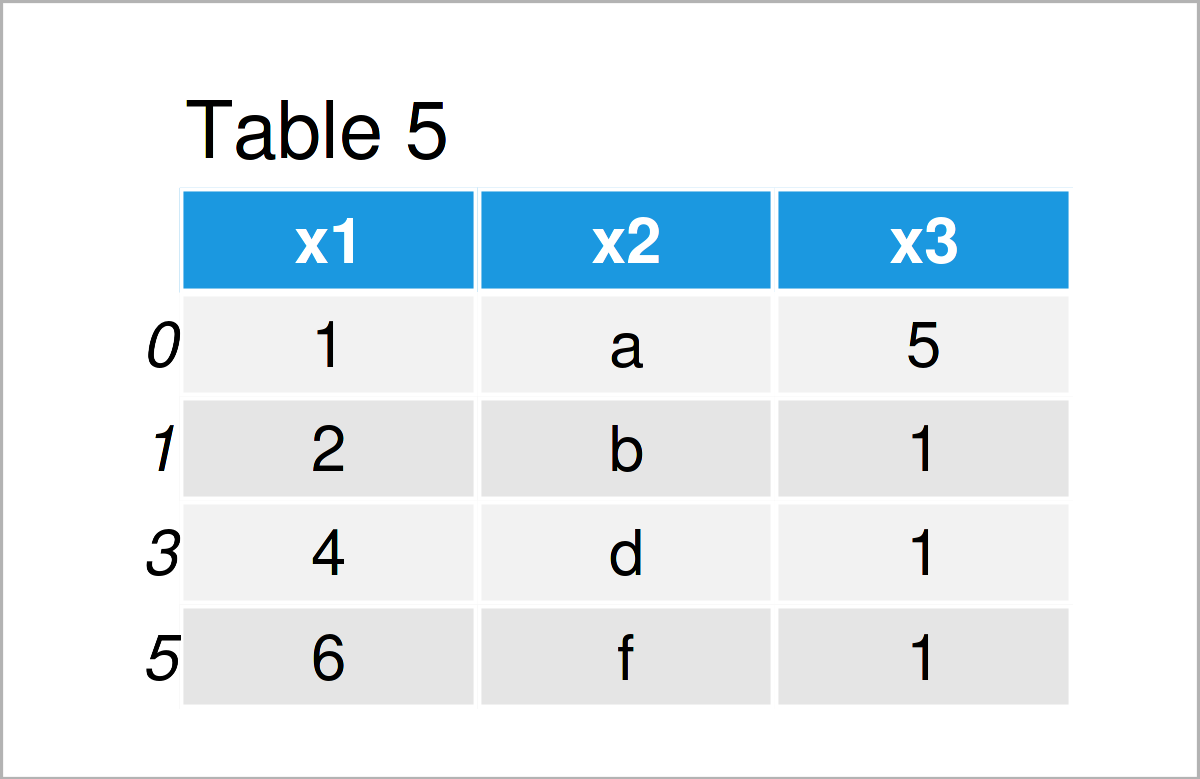# Delete Rows of pandas DataFrame Conditionally in Python (4 Examples)

In this article you’ll learn how to drop rows of a pandas DataFrame in the Python programming language.

The tutorial will consist of this:

Here’s how to do it.

## Example Data & Add-On Packages

First, we have to import the pandas library:

`import pandas as pd                                  # Import pandas library in Python`

Furthermore, consider the example data below:

```data = pd.DataFrame({"x1":range(1, 7),              # Create pandas DataFrame
"x2":["a", "b", "c", "d", "e", "f"],
"x3":[5, 1, 5, 1, 5, 1]})
print(data)                                         # Print pandas DataFrame```As you can see based on Table 1, our example data is a DataFrame and comprises six rows and three variables called “x1”, “x2”, and “x3”.

## Example 1: Remove Rows of pandas DataFrame Using Logical Condition

This example shows how to delete certain rows of a pandas DataFrame based on a column of this DataFrame.

The following Python code specifies a DataFrame subset, where only rows with values unequal to 5 in the variable x3 are retained:

```data1 = data[data.x3 != 5]                          # Using logical condition
print(data1)                                        # Print updated DataFrame```The output of the previous syntax is revealed in Table 2: We have constructed a pandas DataFrame subset with only three rows out of the six input rows.

## Example 2: Remove Rows of pandas DataFrame Using drop() Function & index Attribute

Example 1 has shown how to use a logical condition specifying the rows that we want to keep in our data set.

In this example, I’ll demonstrate how to use the drop() function and the index attribute to specify a logical condition that removes particular rows from our data matrix (i.e. the other way around as in Example 1).

Have a look at the following Python syntax:

```data2 = data.drop(data[data.x3 == 5].index)         # Using drop() function
print(data2)                                        # Print updated DataFrame```Table 3 visualizes the output of the previous Python programming code – The output is exactly the same as in Example 1. However, this time we have used the drop function to create a DataFrame subset.

## Example 3: Remove Rows of pandas DataFrame Using Multiple Logical Conditions

In this example, I’ll demonstrate how to specify different logical conditions for multiple columns to tell Python which rows of our data should be deleted.

The Python syntax below gets rid of all rows where the variable x3 is unequal to 5 and the variable x1 is greater than 2:

```data3 = data[(data["x3"] != 5) & (data["x1"] > 2)]  # Multiple logical conditions
print(data3)                                        # Print updated DataFrame```After running the previously shown Python syntax, the DataFrame subset illustrated in Table 4 has been created.

## Example 4: Remove Rows of pandas DataFrame Based On List Object

So far, we have removed DataFrame rows based on a column of this DataFrame.

This example demonstrates how to drop specific rows of a pandas DataFrame according to the values in a list object (or an array object).

For this example, we first have to create an exemplifying list object in Python:

```my_list = ["yes", "yes", "no", "yes", "no", "yes"]  # Create example list
print(my_list)                                      # Print example list
# ['yes', 'yes', 'no', 'yes', 'no', 'yes']```

The previous output shows the structure of our list: It contains the character strings “yes” and “no”.

Now, we can use this list object to specify a logical condition as basement to subset our data.

The following Python code deletes all lines from our data set, where the corresponding list element of our list object my_list is equal to “no”:

```data4 = data[[x == "yes" for x in my_list]]         # Using list to remove rows
print(data4)                                        # Print updated DataFrame```As shown in Table 5, we have created another pandas DataFrame subset according to the items in our example list.

## Video, Further Resources & Summary

Would you like to learn more about deleting rows of DataFrames using the pandas library? Then you might have a look at the following video that I have published on my YouTube channel. I’m explaining the topics of this post in some more detail.

Please accept YouTube cookies to play this video. By accepting you will be accessing content from YouTube, a service provided by an external third party.If you accept this notice, your choice will be saved and the page will refresh.

In addition, you can have a look at the following video of the Data Science Tutorials YouTube channel. In the video, the speaker explains how to delete rows and columns of a pandas DataFrame.

Please accept YouTube cookies to play this video. By accepting you will be accessing content from YouTube, a service provided by an external third party.If you accept this notice, your choice will be saved and the page will refresh.

In addition, you might read some of the related posts on my website. You can find some articles below.

In summary: You have learned in this tutorial how to remove rows of a pandas DataFrame in the Python programming language. In case you have additional questions, please let me know in the comments section below.

Subscribe to the Statistics Globe Newsletter